# Polynomial in C

## Polynomial in C Language with Examples:

In this article, I am going to discuss Polynomial Representation, Evaluation, and Addition with Examples using C Language. Please read our previous article, where we discussed Array Representation of Sparse Matrix in C and C++ with Examples. At the end of this article, you will learn

1. Polynomial Representation
2. Evaluation of Polynomial
##### Polynomial Representation in C:

We have a polynomial,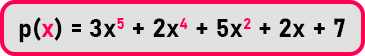It is a collection of terms with a single variable ‘x’. Now let us see how to evaluate a polynomial and perform the addition of polynomials. Once we know addition, we can also learn how to perform subtraction and multiplication of polynomials.

We use polynomial for solving various problems. Various things are represented in the formulas and if the formulas are represented using a single variable, then such formulas are called univariate formulas.

Another problem is we want our programs to use this type of polynomial. Then what programs should be able to evaluate the value of the polynomial. If there is more than one polynomial then our program should be able to add or subtract or multiply them. So first of all, see how the program should represent this polynomial and how we will store this polynomial in our program.

##### Representation of Polynomial:Polynomial is nothing but a collection of terms. In the above polynomial ‘p (x)’, there is a total of 5 terms.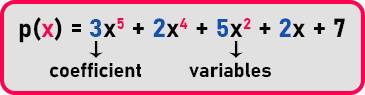Each term of polynomial contains a coefficient, in the above polynomial, ‘3’ is the coefficient of ‘x5’, ‘2’ is the coefficient of ‘x4’, ‘5’ is the coefficient of ‘x2’, ‘2’ is coefficient of ‘x’. And if any term has no coefficient, then it means the coefficient is ‘1’. ‘x’ is a variable with different exponents, i.e., ‘x5’, ‘x4’, ‘x2’ and ‘x’. And if any term doesn’t have a variable the exponent of that variable is ‘0’ i.e., ‘x0’.

We can represent a polynomial as a collection of terms and every term is having a coefficient and exponent. So, we can represent this as a list of terms having coefficients and exponents. So let us prepare a list of 2 things that is coefficients and exponent,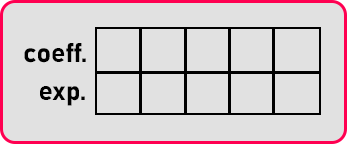Let us fill this table as 3x5 term will fill as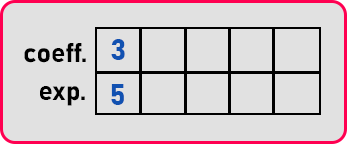In this way, we will fill the whole table.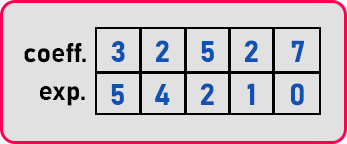Here this table contains a set of terms of the given polynomial. This data is sufficient to represent the above polynomial. One more thing we may want to know that how many non-zero terms are there supports

No. of non-zero terms = length of the polynomial i.e. N = 5

So, this is how polynomial is represented. Now programmatically, how do we present this in a C program? So, for the C program first of all we will define a term, the term is having coefficient and exponent. So, we will define a structure as,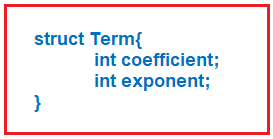Here we have taken coefficient as integer, you can take double or float also depending on your requirement. An exponent is always an integer type. So, we have taken two members and call this structure ‘Term’. I’m calling it a strong. Then what is a polynomial? Polynomial is an array of ‘Terms’. So now let us define the structure for polynomial.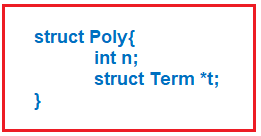This is having two-member, non-zero terms as ‘n’ and pointer of type ‘Term’ to point on the array of ‘Terms’. So, this is a structure for polynomial. It is using the ‘Term’ structure. Now let us learn how to write the program for creating the representation of any polynomial. Here we are writing the pseudocode,

##### Polynomial Representation Pseudo Code: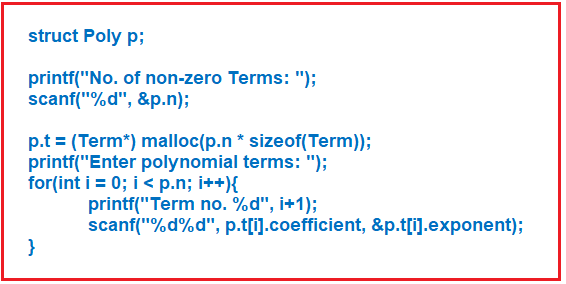Here first we have created an object of type ‘Poly’ that is ‘p’. Next, we are taking input of no. of non-zero terms from the user. Then we allocate the size of the array of terms ‘p.t’.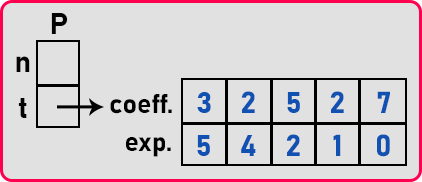Next, we are taking input all the terms as the term’s coefficient and term’s exponent from the user. This is not the complete code. We will see full code in upcoming articles.

##### Polynomial Evaluation in C:

Now, we will see how to evaluate a polynomial. We have a polynomial,In C language, we have implemented the above data as,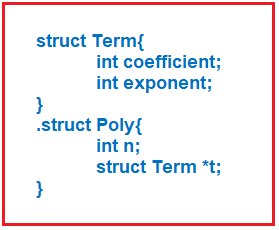1st structure for storing the term data such as coefficient and exponent of term and 2nd structure for creating the polynomial from the array of terms.

##### Representation of Polynomial in C Language: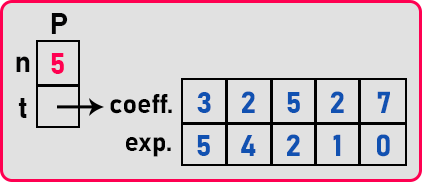Now how to evaluate the polynomial? If we know the value of ‘x’ in the polynomial, then we can get the single value of the answer of the polynomial. First, we have to evaluate all the terms and add them. To evaluate a term, we have to first calculate the exponent part and multiplied with the coefficient. After this, add the result of the first term to the rest of the part of the polynomial.

Every time, we raise ‘x’ to that power and multiplied with the coefficient. All these terms can be computed and added one by one using the ‘for’ loop. So, we have to process them all depending on the number of terms.

##### Polynomial Evaluation Pseudo Code: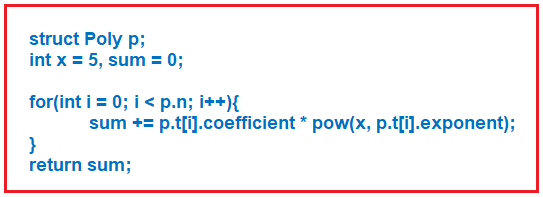Here we have written pseudo code. In this pseudo code, we have declared a variable or object of type ‘Poly’. Next, we have declared the value of ‘x’ as ‘5’ and ‘sum’ as ‘0’. Then we run the ‘for’ loop to add all the terms. In the ‘for’ loop, we multiply the coefficient with the exponent of ‘x’ and store the result in the ‘sum’ variable, and modify the sum in every iteration. In this way, we can evaluate a polynomial.

Now, let us look at how we can add two polynomials and generate a third polynomial. We have our 1st polynomial is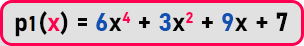We will represent this polynomial data as,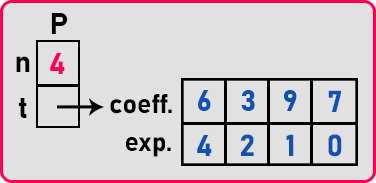The 2nd polynomial is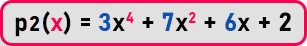We can represent this as,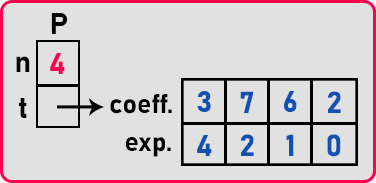Now we want to add these polynomials. For adding these 2 polynomials, we have to create another structure of type ‘Poly’. It will store the result of the addition of polynomials. Representation of 3rd polynomial is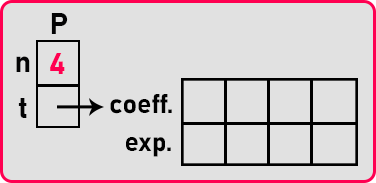So how many terms this polynomial should have? Common exponent terms will be added together and if nothing is common then the size of this polynomial is = total no. of terms of 1st polynomial + total no. of terms of 2nd polynomial.

In this case, the size will be ‘4 + 4 = 8’ if there are no common terms. ‘8’ is the maximum size possible for this polynomial. The number of terms will be less than or equal to ‘8’. Here we know both the polynomials have the same degree terms so we have taken the size ‘4’.

But you have to take the size ‘n1 + n2’ for 3rd polynomial. Because ‘n1 + n2’ maximum that many terms are possible. So, for adding these polynomials, we need an index pointer that points to indices of the array of terms.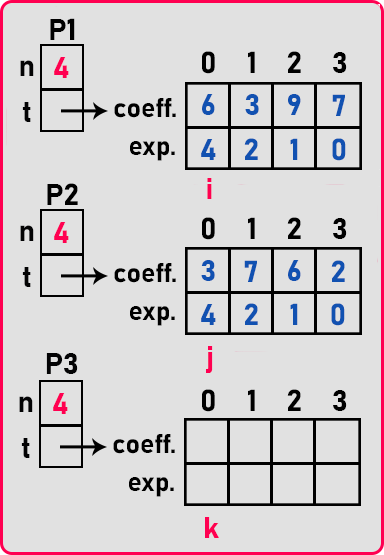We have taken 3 index pointers: ‘i’ for ‘P1’, ‘j’ for ‘P2’, and ‘k’ for ‘P3’. Now let us perform addition. First, we will compare the 1st term of both the polynomials,

P1 = 6x4

P2 = 3x4

Both the terms have the same degree, so add them and add the result to the first term of ‘P3’ as

P3 = 6x4 + 3x4 = 9x4

And now increment all the indices pointer. So now,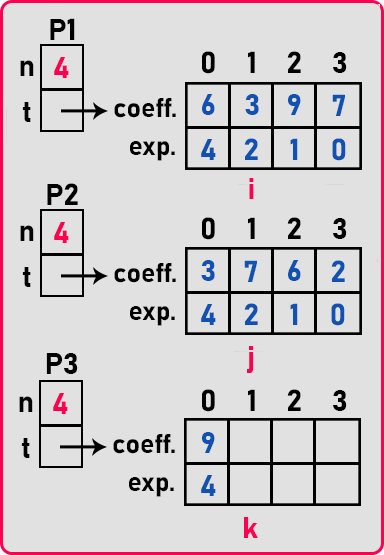Here ‘i’, ‘j’, and ‘k’ are pointing on the 2nd term of all the three polynomials. Now we will compare 2nd term as

P1 = 3x2

P2 = 7x2

Both the terms have the same degree, so add them and add the result to 2nd term of ‘P3’ as

P3 = 3x2 + 7x2 = 10x2

And now increment all the indices pointer. So now,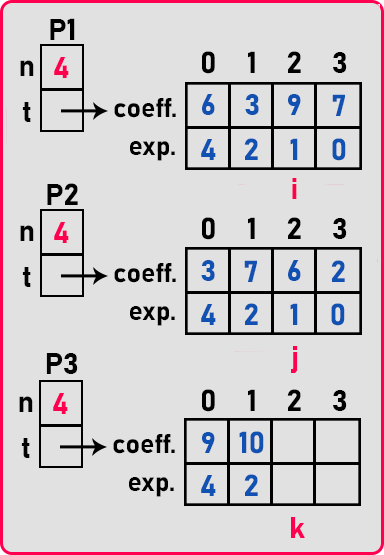So here all the index pointers are pointing on 3rd term of all the polynomials.

Note: Suppose if two terms of two polynomials are not common or their degrees are different then first, we add that term in our 3rd polynomial which has the largest degree, and then increment that largest degree’ polynomial index pointer and other polynomial index pointer remains same.

In this way, we add two polynomials programmatically. The final value of ‘P3’ will be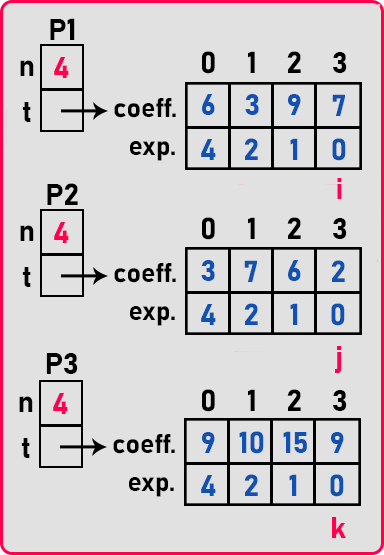‘P3’ will be represented as: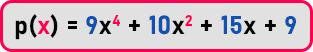In this way, we can perform addition, subtraction, or multiplication on polynomials. Let’s see pseudo-code for this.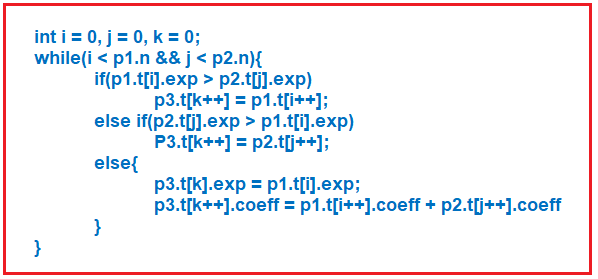##### Polynomial Code in C Language:

Let’s see the C language code for creating a polynomial and performing addition on polynomials

```#include <stdio.h>
#include<stdlib.h>
struct Term
{
int coeff;
int exp;
};

struct Poly
{
int n;
struct Term *terms;
};

void create (struct Poly *p)
{
int i;

printf ("Enter Number of terms: ");
scanf ("%d", &p->n);

p->terms = (struct Term *) malloc (p->n * sizeof (struct Term));

printf ("Enter terms:\n");
for (i = 0; i < p->n; i++)
scanf ("%d%d", &p->terms[i].coeff, &p->terms[i].exp);
printf ("\n");
}

void display (struct Poly p)
{
int i;
for (i = 0; i < p.n; i++)
{
printf ("%dx%d", p.terms[i].coeff, p.terms[i].exp);
if (i + 1 < p.n)
printf (" + ");
}
printf ("\n");
}

struct Poly *add (struct Poly *p1, struct Poly *p2)
{
int i, j, k;
struct Poly *sum;

sum = (struct Poly *) malloc (sizeof (struct Poly));
sum->terms = (struct Term *) malloc ((p1->n + p2->n) * sizeof (struct Term));

i = j = k = 0;

while (i < p1->n && j < p2->n)
{
if (p1->terms[i].exp > p2->terms[j].exp)
sum->terms[k++] = p1->terms[i++];
else if (p1->terms[i].exp < p2->terms[j].exp)
sum->terms[k++] = p2->terms[j++];
else
{
sum->terms[k].exp = p1->terms[i].exp;
sum->terms[k++].coeff = p1->terms[i++].coeff + p2->terms[j++].coeff;
}
}

for (; i < p1->n; i++)
sum->terms[k++] = p1->terms[i];
for (; j < p2->n; j++)
sum->terms[k++] = p2->terms[j];

sum->n = k;
return sum;
}

int main()
{
struct Poly p1, p2, *p3;

printf ("Enter Polynomial 1:\n");
create (&p1);
printf ("Enter Polynomial 2:\n");
create (&p2);

printf ("\n");

printf ("Polynomial 1 is: ");
display (p1);
printf ("\n");

printf ("Polynomial 2 is: ");
display (p2);
printf ("\n");

printf ("Polynomial 3 is: ");
display (*p3);

return 0;
}
```
###### Output: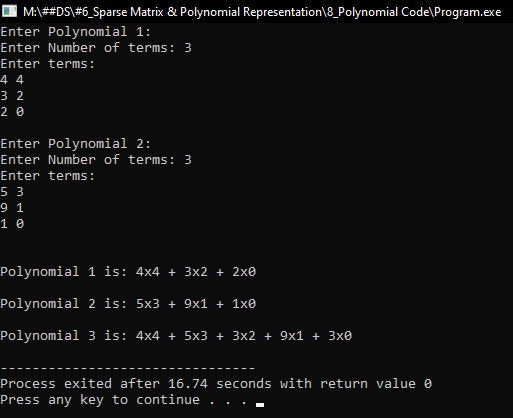In the next article, I am going to discuss why do we need a linked list data structure. Here, in this article, I try to explain Polynomial in C Language with Examples and I hope you enjoy this Polynomial in C Language article.